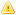Home |Sign in | EnglishHelpWLanguageWLanguage functionsStandard functionsNumeric values management functionsFinancial functionsFinancial functionsRelated examplesSee alsoFinCurrentValFinDecreasingRedemptionFinErrorFinFutureValFinInterestRateFinLinearRedemptionFinNetCurrentValFinPaymentNbFinPeriodInterestFinRedemptionFinRepayValFinancial constantsFinancial functionsThis content has been translated automatically. Click here to view the French version.
WINDEVWEBDEVWINDEV MobileOthersFinancial functions
The financial functions are as follows:
 FinCurrentVal Returns the current value of investment. FinDecreasingRedemption Calculates the amortization value of a good over a specific period of time, via the formula for fixed-rate decreasing amortization. FinError Returns the number of the last error caused by a Finxxx function (financial functions). FinFutureVal Returns the future value of an investment. FinInterestRate Calculates the interest rate for a loan over a specific period of time with fixed payments. FinLinearRedemption Calculates the value of linear amortization of a good over a specific period of time. FinNetCurrentVal Returns the net current value of investment. FinPaymentNb Returns the number of payments required to pay off a capital according to a given rate. FinPeriodInterest Calculates for a given period the amount of interests due for a loan paid off by fixed periodic payments with a fixed interest rate. FinRedemption Creates an amortization matrix for a fixed-rate loan over a defined period of time. FinRepayVal Returns the amount of each regular payment for an investment with fixed interest rate and fixed payment.

Related Examples:Unit examples (WINDEV): The financial functions [ + ] Using the main functions for performing financial calculations in WINDEV:- Calculate the number of repayments- Calculate the future value of an investmentUnit examples (WINDEV): The financial functions [ + ] Using the main functions for performing financial calculations in WINDEV:- Calculate the number of repayments- Calculate the future value of an investmentComplete examples (WINDEV): WD Loan [ + ] The power of WINDEV and the ease-of-use of WLanguage have allowed us to develop this application within a single day!This example is used to simulate loans and to:- calculate the amount of the monthly repayments from the amount borrowed- calculate the amount that can be borrowed from a given monthly repayment- calculate the income of an investment from a monthly paymentFor each case, you have the ability to view the amortization table corresponding to your parameters.Unit examples (WINDEV Mobile): The Financial functions [ + ] Use of financial functions.This example calculates the number of payments needed to pay back a loan, the total cost of a loan as well as the interests returned by an investment.Complete examples (WEBDEV): WW_Loan [ + ] The power of WEBDEV and the simplicity of the WLanguage allowed us to develop this site in just one day!This example is used to simulate loans and to:- calculate the amount of monthly payments from the amount that was borrowed- calculate the amount that can be borrowed from a given monthly payment- calculate the income of an investment from a monthly depositThe amortization table can be displayed for each one of the cases.This table can be edited in a file in PDF format (standard feature of WEBDEV).